Gini Coefficient

The Gini coefficient is a commonly used measure that varies between ‘0’ reflecting complete equality and ‘1’ indicating complete inequality. The Gini coefficient is based on the Lorenz curve, which compares the distribution of incomes across the entire population of an area. It is a useful measure because it incorporates all of the information available from a particular area.

The Gini coefficient of inequality, which is calculated using consumption expenditures per capita, is presented in figure 2.20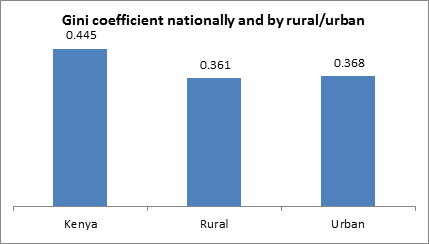Figure 2.20: Gini coefficient nationally and by rural/urban

The national Gini coefficient is estimated at 0.445. This reflects a high level of inequality. The Gini coefficient in rural areas is 0.361 while in urban areas it is 0.368. Thus while inequality in urban areas appears similar to that in rural areas, rural areas have a disproportionately higher population at 68.8 percent compared with 31.2 percent in urban areas and control 45.4 percent of the consumption expenditure. Urban areas control 54.6 percent of consumption expenditure as indicated in figure 2.21. Once we bring them together, however, the inequality levels jump. The leap in national Gini coefficient is due to income gaps between rural and urban areas. A decrease in the income gap between urban and rural areas is therefore a necessary condition for reducing the national Gini coefficient. This is highlighted in the consumption expenditure patterns of rural and urban areas that reflect the prevailing income gaps between the populations in the two areas.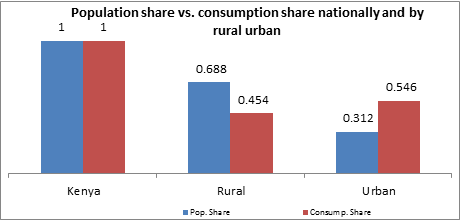Figure 2.21: Population share vs. consumption share nationally and by rural urban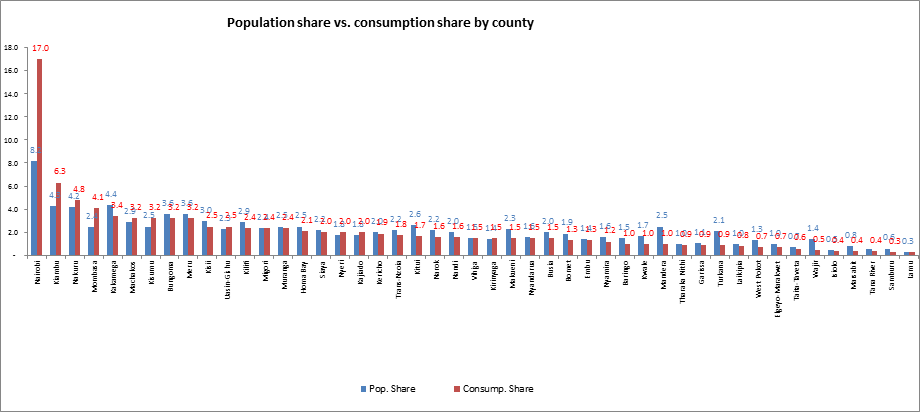Figure 2.22: Population share vs. consumption share nationally and by rural urban

People living in cities and big towns such as Nairobi, Kiambu, Nakuru, Mombasa and Kisumu consume much more than their population share. Nairobi, for example, consumes twice its population share (see figure 2.22).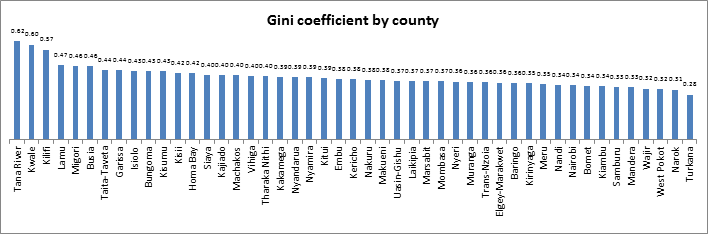Figure 2.23 : Gini coefficient by county

Counties with the greatest inequalities are Tana River, Kwale and Kilifi with Gini coefficients of 0.62, 0.60 and 0.57 respectively (see figure 2.23). These are situated in the Coastal region of the country as seen in figure 2.24. The most equal counties are Turkana, Narok, and West Pokot with Gini coefficients of 0.28, 0.31, and 0.32, respectively. Turkana County emerges as the poorest and the most equal county.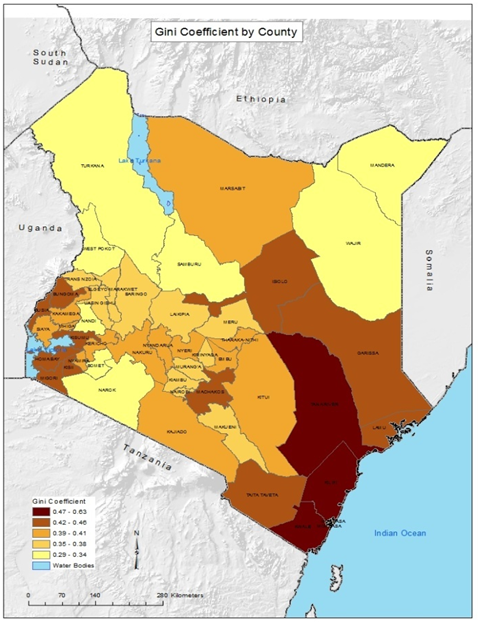Figure 2.24: Gini coefficient nationally